Courses
Courses for Kids
Free study material
Free LIVE classes
More

# Addition Facts for Class 2## Introduction to the Addition Facts

An addition fact is the sum of two specific addends (a number that is added to another) that will always be equal to the same result. For example, 3 + 2 will always be equal to 5. It follows some patterns to add two numbers and write their sum aside.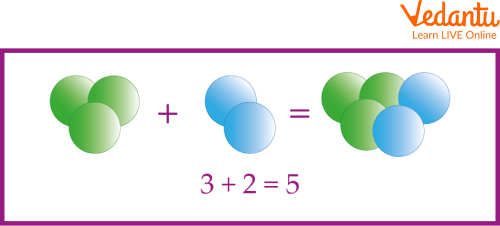Here are some examples of addition facts:

• 7 + 4 = 11 (the addition fact is 11)

• 3 + 6 = 9 (the addition fact is 9)

• 11 + 3 = 14 (the addition fact is 14)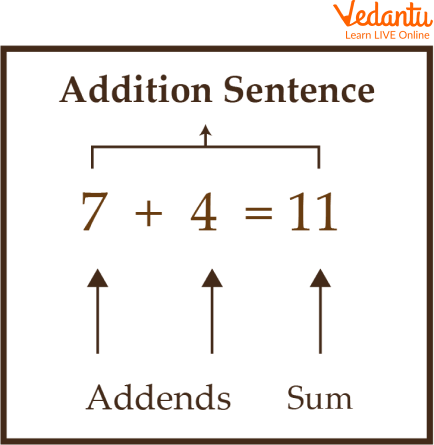## How to Write Addition Facts for Class 2?

You can write addition facts by writing out addition calculations. For example:

• 4 + 11 = 15

• 21 + 8 = 29

• 30 + 14 = 44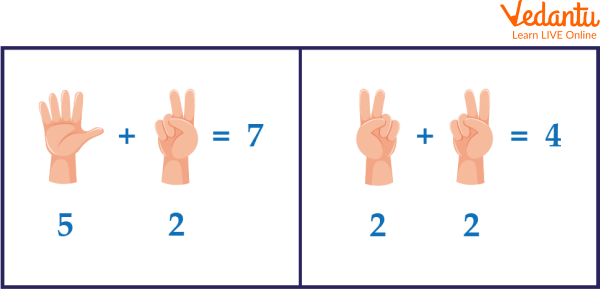Finger Counting

We can also use the number line to add. For example, To add 5 and 7, we can count forward 7 steps from 5, as shown below.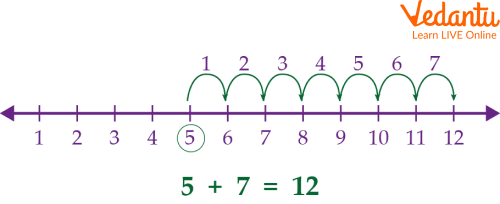Number Line

## How to Learn Addition Facts Chart?

One of the best ways to learn addition facts is through practicing since it helps children to form a good and strong memory of addition facts.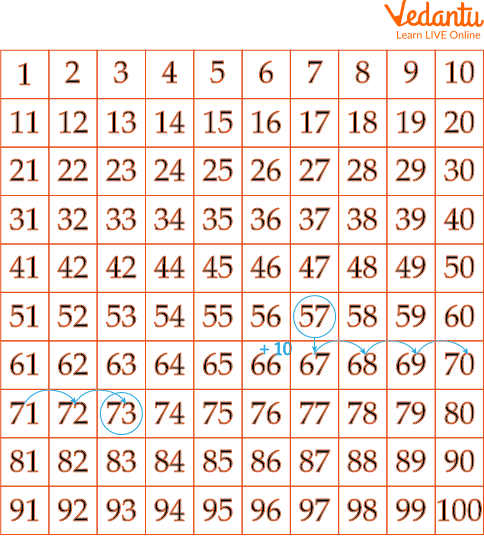A facts chart is a good way to learn addition sums. For example, Add 57 and 16 using addition facts charts.

Step 1: Locate the bigger number (57).

Step 2: If the number that should be added (16) is more than 10, break it into tens and ones, 16 = 10 + 6.
Step 3: Jump as many 10s as in the second number, 57 + 10 = 67.

Step 4: Move forward as many ones as in the second number, 67 + 6 = 73.

So, the sum of 57 and 16 is 73.

Q1. If a bag has 6 balls and 7 more balls are added to it, how many balls are there in the bag?

Ans: Originally, there were 6 balls in the bag. Now 7 more balls have been added.

The number of balls in this bag is now 6 + 7 = 13.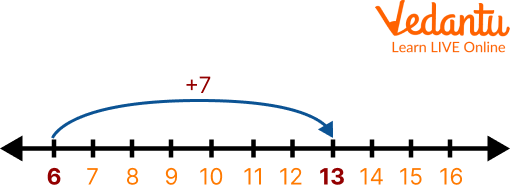Therefore, the bag has 13 balls.

Q2. The present age of Tanvi is 7 years. What will be her age after 10 years?

Ans: Current age of Tanvi is 7 years.

To find Tanvi’s age after 10 years, add 10 to her current age.

After 10 years, her age would be 7 + 10 or 17 years old.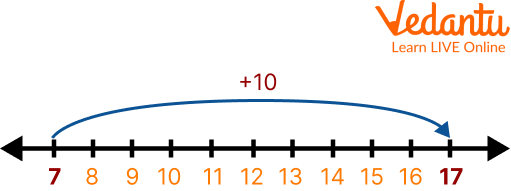Thus the age after 10 years is 17 years.

Q3. Garvita bought a bag for 300Rs and some books for 100Rs. How much did she spend on both?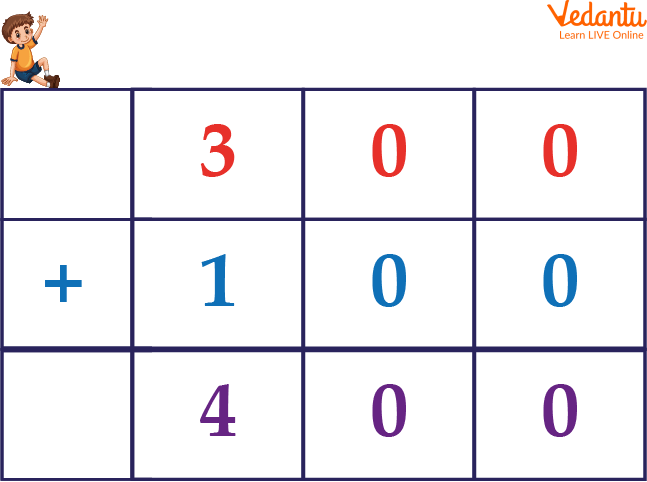Ans: To find the total cost, add 300 and 100.Thus Garvita has spent 400 Rs.

## Practice Questions

Q1. Find the total number of balls in the figure.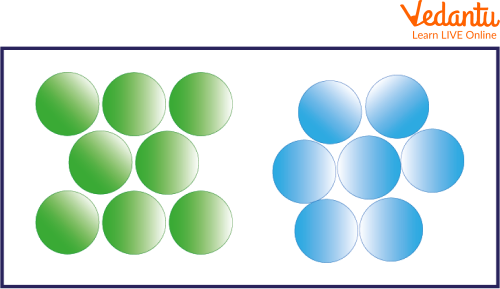Balls

Ans. 15.

Q2. What is the sum of 500 and 127?

Ans. 627.

Q3. Add the numbers and depict the correct sum on the number line.

3 + 6 =?

Ans. 9.

Below given are the addition facts worksheets, which help the students to evaluate their learning of the addition facts.## Summary

In this article, we learned about what are addition facts and some basic facts that are needed for adding two numbers such as the number line and number grid methods and that one of the best ways to learn an addition facts chart is through practising since it helps children to form a good and strong memory of addition facts.

We also learned about how to write and learn addition facts. Some solved and unsolved addition facts examples are also given. Addition facts worksheets serve as a useful tool in evaluating the progress of students.

Last updated date: 01st Oct 2023
Total views: 78.3k
Views today: 1.78k

## FAQs on Addition Facts for Class 2

Addend is the part of the equation where we add two numbers; an addend is defined as the value that is to be added to find a sum.

2. What is meant by addition with and without regrouping?

Addition with regrouping is when the sum of the digits in at least one of the place value columns is greater than 9. Addition without regrouping is when the sum of the digits in each place value column is less than or equal to 9.

3. What are the various properties of addition?

Some basic properties of addition are:

• Closure Property

• Commutative property

• Associative Property

• Distributive Property